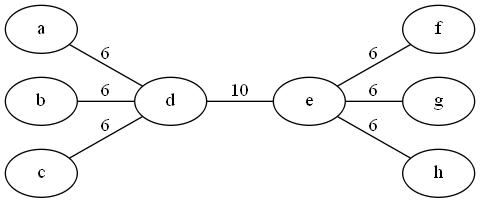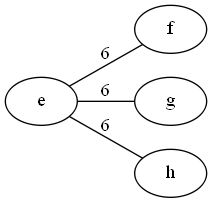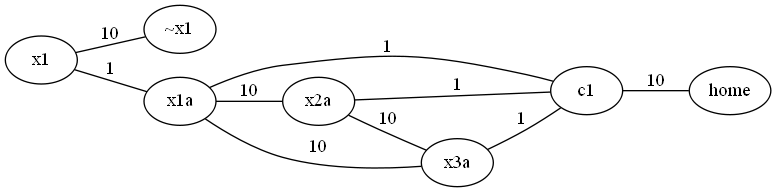Alternating Maximum Weighted Matching

This is a “private communication” problem I haven’t been able to solve or find an actual reduction for.  I think I have the start of a reduction, though, but I haven’t put in the time to work out the details.  Hopefully, I’m on the right track.

The problem: Alternating Maximum Weighted Matching.  This is problem GP8 in the appendix.

The description: Given a weighted graph G=(V,E), with positive weights, and a positive bound B, each player chooses an edge from E.  No two chosen edges can share an endpoint.  Player 1 wins if the weights of the edges chosen ever exceed B.  Does player 1 have a forced win?

Example: Here’s a graph:If B=11, and it’s player 1’s turn, then they can’t remove the highest-cost edge (d,e) because every edge is incident on d or e, so player 2 would have no moves, and the game would end with cost 10.

If player 1 removes one of the 6-cost edges (let’s say (a,d)) we’re left with:..and so player 2 will have to take one of the remaining 6-cost edges, bringing the total cost of edges removed to 12.

Reduction (sort of): So, like I said at the top, the reference in G&J is to a “private communication” by Dobkin and Ladner.  I couldn’t find the actual result published anywhere.  I actually emailed both Dobkin and Ladner to ask if they remembered what the reduction was, but their response (reasonably) was “It’s been 40 years, I have no idea”.

But, I thought about it for a little while, at least, and came up with what I thought are the beginnings of an idea.  I didn’t have the time (or, perhaps, the ability) to get all of the details right, but this feels like a start, at least:

We’re going to reduce from One in Three 3SAT.  Each variable will be represented as a pair of vertices xi and xia, connected by a weight 1 edge.  The negation of the variable (~xi and ~xia) will also be vertices and also connected by a weight 1 edge.  The vertices xi and ~xi will be connected by a “large” edge of weight 10.

From each clause, we build up a component of a graph that looks like this:The weights of 10 and 1 might not be right, think of them as “large” and “small” weights.  Each of the xi variables corresponds to the actual variables in the formula, we only include the variables that correspond to the clause we’re looking at.

(One thing I did wrong was to assume that player 1 loses if the edge cost goes over B.  So in what follows, player 1 is trying to keep the score low.  We can fix this by making it player 2’s turn and swapping the roles)

The idea is that player 1 will choose either the x1-x1a edge or the ~x1-~x1a edge to “fix” the value of the first variable.  If the variable shows up in the clause (for example, they chose the x1-x1a edge in the diagram above), this will eliminate the edges (x1a,c1), (x1a, x2a), and (x1a, x3a) from being able to be chosen.

Then player 2 will want to choose an “expensive” edge.  He’ll choose the edge (x2a,x3a)

Then we’ll move on to the next variable.  It’s again player 1’s turn to decide on a setting of x2.

The idea is that each clause will have it’s “ci” vertex connect to that central “home” vertex by an expensive edge, so if after all of the variables have been given their values, there still is an edge to the home vertex, it will be chosen, and that amount will be the amount that sends the total cost over the bound.  (So right now, I’m thinking of the bound being something like 11*N +1 for a problem with N variables, at least until the extra things below get added).

What still needs to be done is the detail work (and probably extra edges and vertices) to ensure that players have to choose the edges in the order I specify (i.e., not doing so loses a player the game immediately).  It’s entirely possible that doing so will make this whole construction wrong.  But I like the idea behind it, at least

Difficulty: N/A.  I don’t want to call this a 10, even though it stumped me.  I think if my idea is right, it’s not that hard.